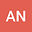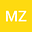loading page

A numerical approach based on $n$-dimensional fractional M\”{u}ntz-Legendre polynomials for solving fractional-order cohomological equations with variable coefficients
••Mina Moghaddam Zeabadi
Iran University of Science and Technology
Author Profile## Abstract

This research presents a numerical approach to obtain the approximate solution of the n-dimensional cohomological equations of fractional order in continuous-time dynamical systems. For this purpose, the $n$-dimensional fractional M\”{u}ntz-Legendre polynomials (or n-DFMLPs) are introduced. The operational matrix of the fractional Riemann-Liouville derivative is constructed by employing n-DFMLPs. Our method transforms the cohomological equation of fractional order into a system of algebraic equations. Therefore, the solution of that system of algebraic equations is the solution of the associated cohomological equation. The error bound and convergence analysis of the applied method under the $L^{2}$-norm is discussed. Some examples are considered and discussed to confirm the efficiency and accuracy of our method.

#### Peer review status:UNDER REVIEW

09 Mar 2021Submitted to Mathematical Methods in the Applied Sciences
11 Mar 2021Assigned to Editor
11 Mar 2021Submission Checks Completed
13 Mar 2021Reviewer(s) Assigned